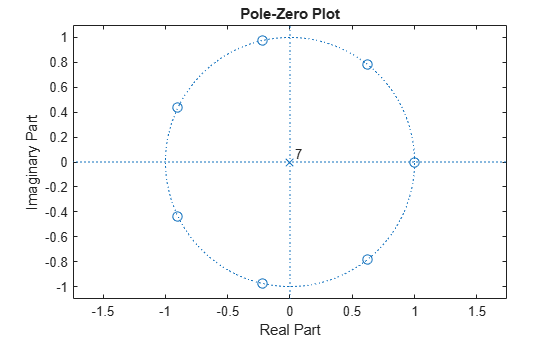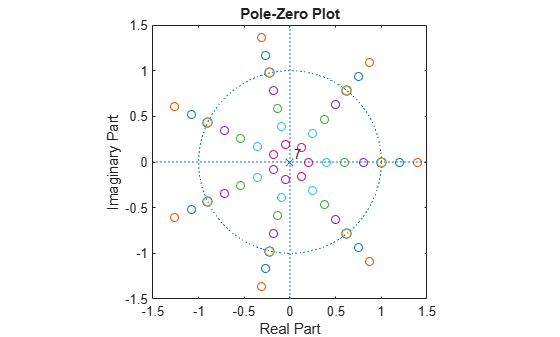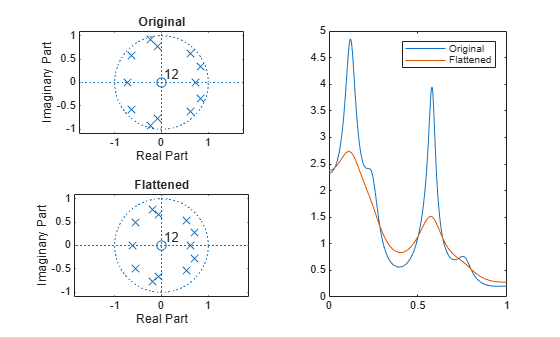Documentation

# polyscale

Scale roots of polynomial

## Syntax

```b = polyscale(a,alpha) ```

## Description

`b = polyscale(a,alpha)` scales the roots of a polynomial in the z-plane, where `a` is a vector containing the polynomial coefficients and `alpha` is the scaling factor.

If `alpha` is a real value in the range `[0 1]`, then the roots of `a` are radially scaled toward the origin in the z-plane. Complex values for `alpha` allow arbitrary changes to the root locations.

## Examples

collapse all

Express the solutions to the equation ${x}^{7}=1$ as the roots of a polynomial. Plot the roots in the complex plane.

```pp = [1 0 0 0 0 0 0 -1]; zplane(pp,1)```Scale the roots of `p` in and out of the unit circle. Plot the results.

```hold on for sc = [1:-0.2:0.2 1.2 1.4]; b = polyscale(pp,sc); plot(roots(b),'o') end axis([-1 1 -1 1]*1.5) hold off```Load a speech signal sampled at ${F}_{s}=7418\phantom{\rule{0.2777777777777778em}{0ex}}Hz$. The file contains a recording of a female voice saying the word "MATLAB®."

`load mtlb`

Model a 100-sample section of the signal using a 12th-order autoregressive polynomial.

```Ao = lpc(mtlb(1000:1100),12); Ax = polyscale(Ao,0.85);```

Perform bandwidth expansion of the signal by scaling the roots of the autoregressive polynomial by 0.85. Plot the zeros, poles, and frequency responses of the models.

```subplot(2,2,1) zplane(1,Ao) title('Original') subplot(2,2,3) zplane(1,Ax) title('Flattened') subplot(1,2,2) [ho,w] = freqz(1,Ao); [hx,w] = freqz(1,Ax); plot(w/pi,abs([ho hx])) legend('Original','Flattened')```## Tips

By reducing the radius of the roots in an autoregressive polynomial, the bandwidth of the spectral peaks in the frequency response is expanded (flattened). This operation is often referred to as bandwidth expansion.